### Background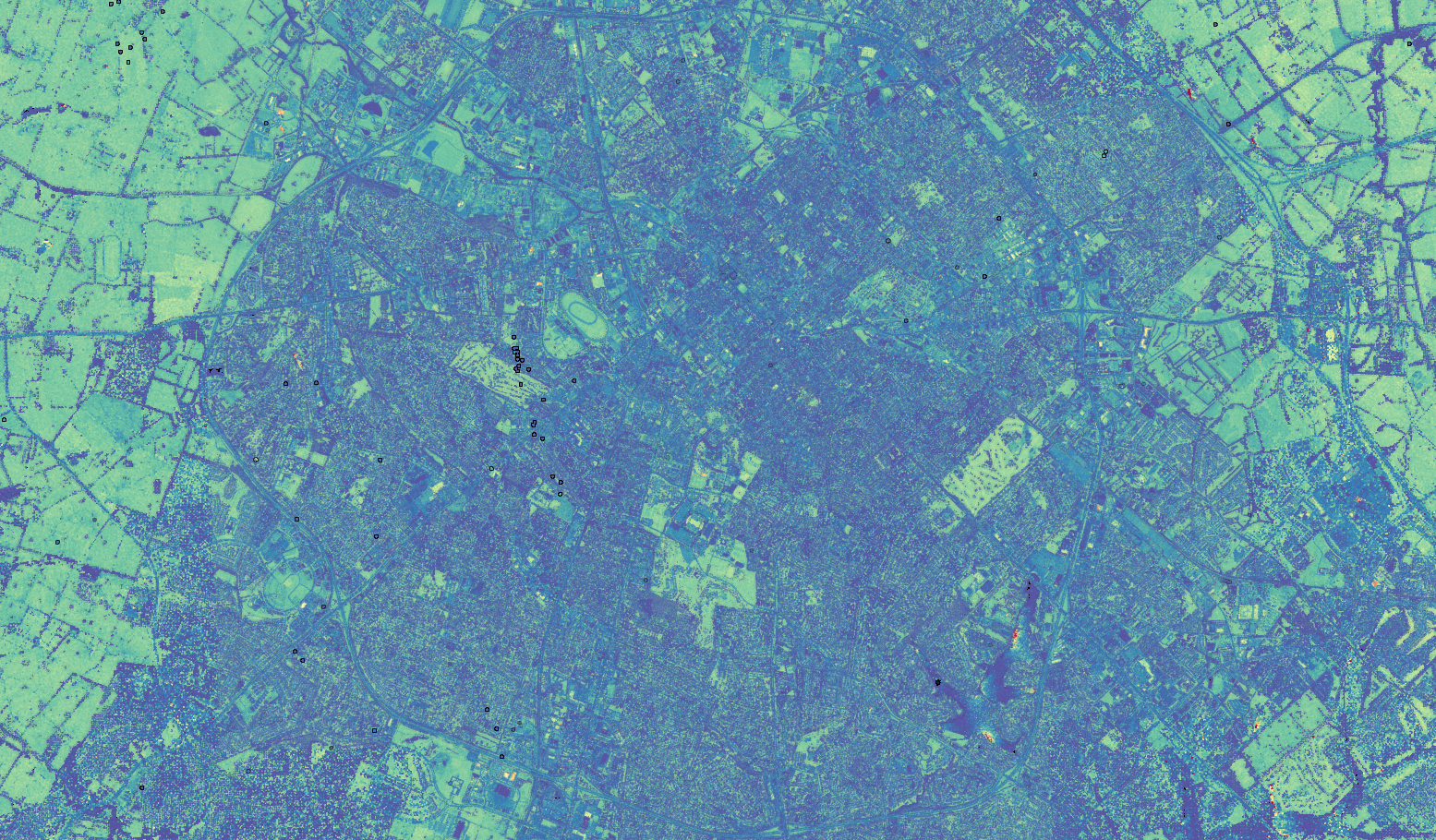Lexington, KY from aboveFrankfort, KY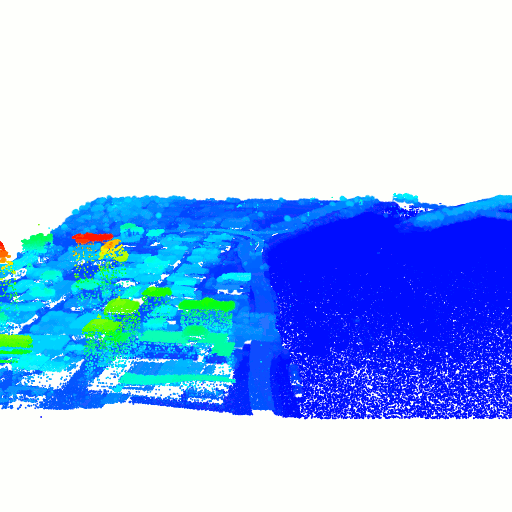Louisville, KY

LiDAR is a way to capture 3D scenes, akin to photographs capturing 2D scenes. The data itself has some attributes such as $$x$$, $$y$$, and $$z$$ values representing a point in space, along with some other metadata like GPS time (when the point was captured), scan angle (the angle between the LiDAR sensor and the captured data point), etc. When viewing the points, the result is a point cloud which can be colored by whatever feature you want, e.g. time or intensity. However, depending on the data collection specifications, some features might not be included. In the case of the Kentucky LiDAR data, the scan angle is not included. Moreover, features such as intensity seem to be dependent on the scan angle. To account for this variation, estimating the scan angle, and hence reconstructing the flight route of the LiDAR sensor, is crucial.

### ApproachesComputed scan direction vectors for a flight over Frankfort, KY

One of the key features included in LiDAR data is the number of returns. Essentially, whenever a pulse from the LiDAR sensor passes through a semi-transparent material (such as a leaf), that will register a return and this process continues until the pulse hits bare earth. These returns are then recorded as separate data points, but can be grouped together via the time or the scan angle. To reconstruct the flight route of the LiDAR sensor, working with the times with multiple returns is a good start. Using the times with multiple returns, the scan angle can be recovered by computing a difference vector between two points with the same timestamp as follows: $\mathbf{d}_t = \frac{P_1 - P_2}{\lvert\lvert P_1 - P_2 \rvert\rvert},$ $\mathbf{d}_t = \begin{cases} \mathbf{d}_t & \text{if } d_z \geq 0 \\ -\mathbf{d}_t & \text{if } d_z < 0 \end{cases}$ This gives us a normalized scan direction vector that is oriented toward the direction of the flight route. Now for each time where multiple returns are recorded, there is a scan direction vector that gives an indication of the LiDAR sensor's flight route.

1. #### Geometry

2.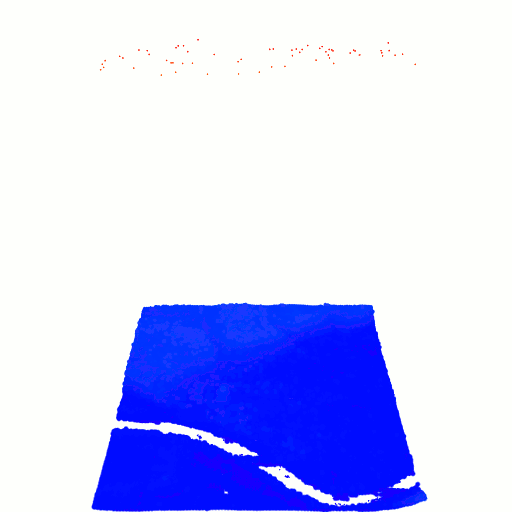Estimate of flight over Frankfort, KY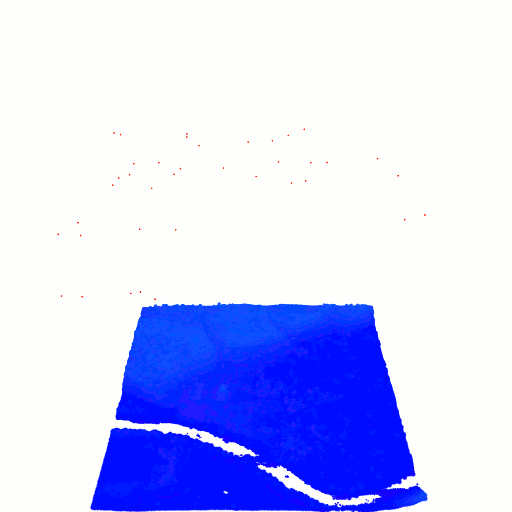Estimate of multiple flights over Frankfort, KY

Let $$T$$ be the sorted list of times. Given a scan direction vector $$\mathbf{d}_i$$ corresponding to some data point $$P_i$$, where $$i$$ is the index into the list of times, we first paramaterize the line formed by the scan direction vector and the data point by $$\alpha_i = \delta \mathbf{d}_i + P_i$$. Due to the constraints of the physical world, we can extend this line to some large distance, $$0 \leq \delta \leq 8,000$$. To discretize this line, we simply make $$n$$ equidistant points on the line. For each pair $$i, i+1$$, we then compute a pairwise distance matrix between the discretized lines and find the points with minimal distance: $\DeclareMathOperator*{\argmin}{arg\,min} % Jan Hlavacek j,k = \argmin_{d\left(\alpha_{i_j}, \beta_{(i+1)_k}\right)} \begin{bmatrix} d\left(\alpha_{i_0}, \beta_{(i+1)_0}\right) & d\left(\alpha_{i_0}, \beta_{(i+1)_1}\right) & \cdots & d\left(\alpha_{i_0}, \beta_{(i+1)_n}\right) \\ d\left(\alpha_{i_1}, \beta_{(i+1)_0}\right) & d\left(\alpha_{i_1}, \beta_{(i+1)_1}\right) & \cdots & d\left(\alpha_{i_1}, \beta_{(i+1)_n}\right) \\ \vdots & \vdots & \ddots & \vdots \\ d\left(\alpha_{i_n}, \beta_{(i+1)_0}\right) & d\left(\alpha_{i_n}, \beta_{(i+1)_1}\right) & \cdots & d\left(\alpha_{i_n}, \beta_{(i+1)_n}\right) \end{bmatrix},$ where $$j,k$$ are the indices into the sets of discretized lines $$\{\alpha_{i_j}\}, \{\beta_{(i+1)_k}\}$$. This will then give us the two nearest points along two (adjacent) scan direction vectors. After iterating over all (adjacent) pairs of times, this process will yield a collection of points that approximate the flight route, as shown in the images above.

3. #### Linear Motion Model

4.
Estimated linear flight route over Frankfort, KY

If we assume that flight route of the LiDAR sensor is linear, then we can use the scan direction vectors to find a line that minimizes the distance between the scan direction vectors and the line. In this way, we can estimate a function $$f(t) = At + \mathbf{t}$$ where $$A$$ is a learnable $$1 \times 3$$ matrix representing the initial direction of the flight route and $$\mathbf{b}$$ is a learnable $$1 \times 3$$ bias term that represents the initial position of the LiDAR sensor. Using the optimization library PyTorch, we can create a model that predicts a scan direction vector given a time. We can then set up our objective function by minimizing the cosine distance between the computed scan direction vector and the predicted scan direction vector: $\min_{\theta} \sum_t \frac{\mathbf{d}_t (f(t, \theta) - X_t)}{\lvert\lvert f(t, \theta) - X_t \rvert\rvert},$ where $$\theta$$ are the parameters of the model, $$t$$ are times, $$\mathbf{d}_t$$ are the computed scan direction vectors, $$f(t, \theta)$$ is the model prediction, and $$X_t$$ are the ground constaints (data points). By using this formulation, the model effectively learns to predict a point along the linear flight route that also matches the computed scan direction vector. The results seem better than the geometric approach, but are also more restrictive since we assume the motion model to be linear.